# C++ Program to Count Characters, Words, and Lines in a File

This article provides some programs in C++ that count and print the total number of characters, words, lines, vowels, etc. in a file. The file's name must be entered by the user at run-time. This article deals with these programs:

#### What Should You Do Before the Program?

Since the program given below operates on a file. Therefore, a file must be available or created inside the current directory (the folder where the C++ program's source code is saved). I haven't created a file, so let me create a file called codescracker.txt. Here is a snapshot of the folder where this file was created and saved right now:Now put some content inside this file so that the program given below counts all the things like characters, words, lines, vowels, consonants, blank spaces, etc. available in the file. Here is a snapshot of the opened file, codescracker.txt, after writing or adding some content: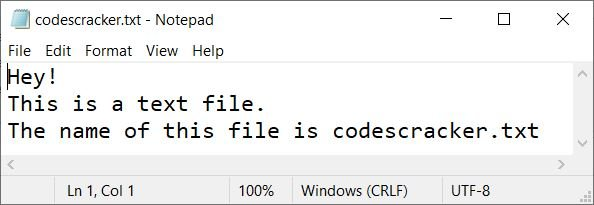Now let's move on and create a program in C++ that counts characters, words, lines, etc. from this file using the program.

## Count the Number of Characters in a File

The question is, "Write a program in C++ that receives the name of a file from the user at run-time to count and print the total number of characters available in the file." The answer to this question is the program given below:

```#include<iostream>
#include<fstream>

using namespace std;
int main()
{
char filename, ch;
int tot=0;
ifstream fp;
cout<<"Enter the Name of File: ";
cin>>filename;
fp.open(filename, ifstream::in);
if(!fp)
{
return 0;
}
while(fp>>noskipws>>ch)
tot++;
fp.close();
cout<<endl<<"Total Characters = "<<tot;
cout<<endl;
return 0;
}```

The snapshot given below shows the initial output produced by the above C++ program on counting the total number of characters available in a text file: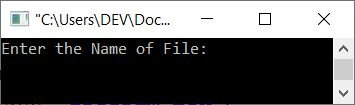Now enter the name of the file, say codescracker.txt (the file created earlier), and press the ENTER key to count and print the total number of characters as shown in the snapshot given below: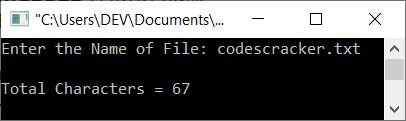Note: The noskipws is used in between the file pointer fp and the character variable ch while scanning or getting the character, so as to extract and initialize all the things (i.e., the whole content of the file), including tab spaces, blank spaces, newlines, etc.

## Count the Number of Words in a File

This program counts and prints the total number of words available in a file entered by the user. Except for the counting part, the majority of the code will be the same as in the previous program. Let's have a look at the program given below:

```#include<iostream>
#include<fstream>

using namespace std;
int main()
{
char filename, ch, str;
int tot=0, i=0, tot_word=0;
ifstream fp;
cout<<"Enter the Name of File: ";
cin>>filename;
fp.open(filename, ifstream::in);
if(!fp)
{
return 0;
}
while(fp>>noskipws>>ch)
{
str[tot] = ch;
tot++;
}
fp.close();
str[tot]='\0';
while(str[i]!='\0')
{
if(str[i]=='\n')
{
if(str[i+1]!='\0' && str[i+1]!=' ')
tot_word++;
i++;
}
else if(str[i]!=' ')
i++;
else
{
if(str[i+1]!='\0' && str[i+1]!=' ')
tot_word++;
i++;
}
}
cout<<endl<<"Total Words = "<<tot_word;
cout<<endl;
return 0;
}```

Here is its sample run with the same user input, which is codescracker.txt as the name of the file:In the above program, the statement:

`str[tot]='\0';`

is used to end the initialization of a character in string str with a null character ('0'). And the following block of code:

```   while(str[i]!='\0')
{
if(str[i]=='\n')
{
if(str[i+1]!='\0' && str[i+1]!=' ')
tot_word++;
i++;
}
else if(str[i]!=' ')
i++;
else
{
if(str[i+1]!='\0' && str[i+1]!=' ')
tot_word++;
i++;
}
}```

is responsible for counting the total number of words available in the string str. This string contains all the content of the file. Therefore, indirectly, the code is used to count the total number of words in the file. In this block of code, the code:

`while(str[i]!='\0')`

states that the loop will continue to execute until a null-terminated character ('\0') is encountered. And the code:

`if(str[i+1]!='\0' && str[i+1]!=' ')`

states that the condition evaluates to be true only if the character at i+1th index is not equal to a null terminated character and also not equal to a blank space.

## Count the number of lines in a file

This is, I think, the simplest one of all the programs given here. The only extra thing we've got to do in this program is only compare the character at the current index with \n and increment the variable responsible for the counting of newlines or the number of lines available in the file, like shown in the program given below:

```#include<iostream>
#include<fstream>

using namespace std;
int main()
{
char filename, ch, str;
int tot=0, i=0, tot_lines=0;
ifstream fp;
cout<<"Enter the Name of File: ";
cin>>filename;
fp.open(filename, ifstream::in);
if(!fp)
{
return 0;
}
while(fp>>noskipws>>ch)
{
str[tot] = ch;
tot++;
}
fp.close();
str[tot]='\0';
while(str[i]!='\0')
{
if(str[i]=='\n')
tot_lines++;
i++;
}
tot_lines++;
cout<<endl<<"Total Number of Lines = "<<tot_lines;
cout<<endl;
return 0;
}```

Here's a sample run with user input codescracker.txt to count and print the total number of lines in this file, as shown in the screenshot below: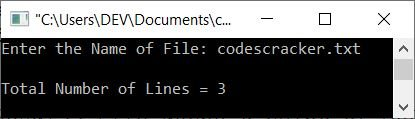## Total number of blank spaces in the file

This program is as simple as the previous program. Here also, we only have to compare each character with ' ' a blank space, one by one, as shown in the program given here:

```#include<iostream>
#include<fstream>

using namespace std;
int main()
{
char filename, ch, str;
int tot=0, i=0, tot_blanks=0;
ifstream fp;
cout<<"Enter the Name of File: ";
cin>>filename;
fp.open(filename, ifstream::in);
if(!fp)
{
return 0;
}
while(fp>>noskipws>>ch)
{
str[tot] = ch;
tot++;
}
fp.close();
str[tot]='\0';
while(str[i]!='\0')
{
if(str[i]==' ')
tot_blanks++;
i++;
}
cout<<endl<<"Total Number of Blank Spaces = "<<tot_blanks;
cout<<endl;
return 0;
}```

Here is a snapshot of the sample run with the same user input as the previous program, which is codescracker.txt: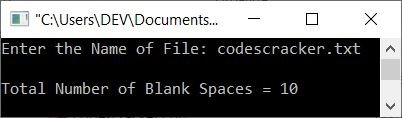## Count vowels and consonants in the file

This is the final program in this article, and it counts and prints the total number of vowels and consonants in a text file:

```#include<iostream>
#include<fstream>

using namespace std;
int main()
{
char filename, ch, str;
int tot=0, i=0, vowels=0, consonants=0, ascii;
ifstream fp;
cout<<"Enter the Name of File: ";
cin>>filename;
fp.open(filename, ifstream::in);
if(!fp)
{
return 0;
}
while(fp>>noskipws>>ch)
{
str[tot] = ch;
tot++;
}
fp.close();
str[tot]='\0';
while(str[i]!='\0')
{
if(str[i]=='a'||str[i]=='e'||str[i]=='i'||str[i]=='o'||str[i]=='u')
vowels++;
else if(str[i]=='A'||str[i]=='E'||str[i]=='I'||str[i]=='O'||str[i]=='U')
vowels++;
else
{
ascii = str[i];
if(ascii>=65 && ascii<=90)
consonants++;
else if(ascii>=97 && ascii<=122)
consonants++;
}
i++;
}
cout<<endl<<"Total Number of Vowels = "<<vowels;
cout<<endl<<"Total Number of Consonants = "<<consonants;
cout<<endl;
return 0;
}```

The snapshot given below shows a sample run of the above program on counting the total number of vowels and consonants in a text file entered by the user at run-time:The above program is created in such a way that first I've checked whether the character is a vowel or not. If not, then using the else block, the character gets initialized to a variable of type int that assigns the ASCII equivalent of the character, and using the ASCII value, I've done the thing.

Note that the ASCII values for letters A-Z are 65–90 and for letters a–z are 97–122, respectively.

You can also replace the following block of code from the above program:

```      else
{
ascii = str[i];
if(ascii>=65 && ascii<=90)
consonants++;
else if(ascii>=97 && ascii<=122)
consonants++;
}```

with the block of code given below:

```      else
{
if(str[i]>='A' && str[i]<='Z')
consonants++;
else if(str[i]>='a' && str[i]<='z')
consonants++;
}```

The results will be the same. I've provided both variety and context to ensure that you have a thorough understanding of the subject.

C++ Quiz

« Previous Program Next Program »